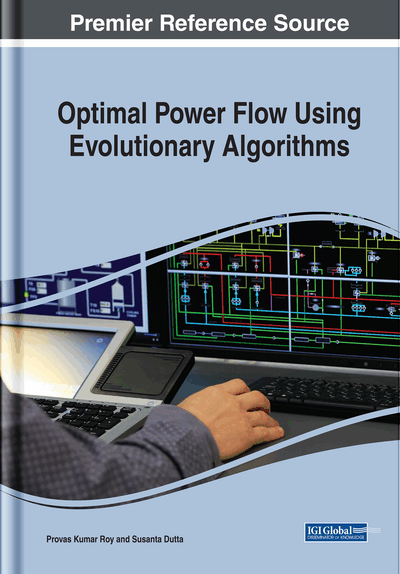# Economic Load Dispatch: Optimal Power Flow and Optimal Reactive Power Dispatch Concept

DOI: 10.4018/978-1-5225-6971-8.ch002
Available
\$37.50
No Current Special Offers

## Abstract

The main objective of the power system is to deliver electric energy to its loads economically and efficiently in a safe and reliable manner. Due to the complicated structure of the present power system network and competitive environment introduced by deregulation, optimal power flow (OPF) and optimal reactive power flow (ORPD) provide efficient exploitation of existing power generations. This chapter describes the detail problem formulation of OPF and ORPD problems. In this study, three different single objectives, namely fuel cost minimization, voltage profile improvement, and transmission loss minimization, are considered. Moreover, in order to judge the effectiveness of the proposed methods for multi-objective scenario, two bi-objectives, namely simultaneous minimization of fuel cost and voltage deviation; simultaneous minimization of fuel cost and transmission loss; and one tri-objective function, namely simultaneous minimization of fuel cost with voltage deviation and loss, are considered.
Chapter Preview
Top

The objective of ELD (Qin et al., 2017) is to minimize the generation cost while satisfying all the equality and inequality constraints and is given by (1)Minimize(1) where,is the total fuel cost;,are the fuel cost and generating power, respectively, of the ith generating unit; n is the number of generators.